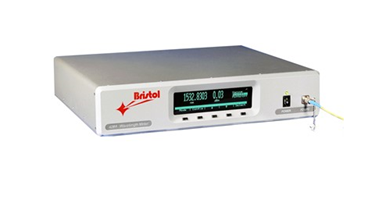# 428 Series Multi-Wavelength MeterThe 428 Multi-Wavelength Meter combines proven Michelson interferometer-based technology with fast Fourier transform analysis in order to measure wavelength, power and OSNR of as many as 1000 discrete optical signals. Wavelength is measured to an accuracy as high as ± 0.3 pm and power is measured to an accuracy of ± 0.5 dB. In addition, the 428 system automatically calculates OSNR to greater than 40 dB.

Features include:

• Simultaneously measures wavelength and power of up to 1000 discrete optical signals
• Wavelength measured to an accuracy as high as ± 0.3 pm
• Continuous calibration with a built-in wavelength standard
• Measurement confidence level ≥ 99.7%
• Traceable to NIST standards
• Power measured to an accuracy of ± 0.5 dB
• Automatically calculates OSNR to > 40 dB
• Operates with CW and modulated signals
• Rugged design for manufacturing environments